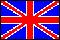ISSN 2079-3537

Scientific Visualization
 Issue Year: 2015 Quarter: 1 Volume: 7 Number: 1 Pages: 65 - 77
 Article Name: MATHEMATICAL MODELING AND VISUALIZATION OF INTRACHAMBER PROCESSES IN A BALLISTIC SETUP WITH HYDRODYNAMIC EFFECT Authors: N.V. Bykov (Russian Federation), E.A. Nesterenko (Russian Federation) Address: N.V. Bykov bykovnv@bk.ru Bauman Moscow State Technical University, Russia Dorodnicyn Computing Centre of RAS, Russia E.A. Nesterenko nesterenko_ea@bk.ru Bauman Moscow State Technical University, Russia Keldysh Institute of Applied Mathematics RAS, Russia Abstract: The questions of mathematical modeling and visualization of the results of intrachamber processes in ballistic systems with hydrodynamic effect numerical calculation were considered. Hydrodynamic effect allows one to receive a higher rate of muzzle velocity by accelerating the deformable piston front as it passes through a tapered conical section. A mathematical model of the shot process, taking into account the two-phase character of gas-powder mixture motion and viscoplastic properties of a deformable piston is constructed. Numerical solution of the problem is carried out using a modified method of Godunov type finite volume on a moving mesh. Since the considered system of equations, describing the intrachamber processes has areas in which it loses its hyperbolicity, in determining of the flow through the cell boundaries two different schemes were used: AUSM+ to calculate the flows of the gas phase and in the field of deformable piston, and Rusanov scheme to calculate the flows in the condensed phase. Due to the use of moving mesh particular attention is given to visualization the numerical calculation results, since the data is located on unstructured grids. Two methods of visualization of the data were considered: the animation of the scalar field and a two-dimensional representation of the density of scalar fields. It is shown that each of the methods has its own advantages and disadvantages. Language: Russian